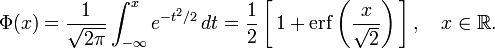## Tuesday, March 27, 2012

### More About Natural Heat Waves

So what is the natural rate of temperature extremes?

Here's what I was thinking about the chances of finding extreme weather events somewhere on Earth, in the absence of climate change, though I don't have it all figured out. I'll use some simple math because it makes it easier to think.

Let E be the Earth's surface area (510 million km2), and divide the surface into regions all of area A. The number of such regions on the planet is E/A.

Let D be the length, in days, of your daily temperature measurements. In D days the number of measurements for the entire planet is D*(E/A).

We want to consider temperature fluctuations, whose length is some number of days N.

For any region, its daily "average temperature" is the average of the daily high and daily low (as meteorologists often do). The N-day average as just the average of daily average temperatures of the last N days. Subtract the mean for those days, and call this the "N-day fluctuation," as we do, and to save writing call it FN.

There will be 365.25 of these fluctuations every year, for every N -- some below zero, some above zero. They have some distribution, and some chance of being more than 3 standard deviations above the mean, or 5 standard deviations below the mean, etc.

What is the chance one of these fluctuations is "extreme" -- more than σ standard deviations above the mean? It's defined by

p(σ) = 1/[D*(E/A)]

where p is the probability of the fluctuation. So if you pick the size of the region and the extremeness of the fluctuation, and you know the probability distribution, you can calculate the average number of days between them.

Assume all these fluctuations are Gaussian-distributed. They may not be, according to Coumou and Rahmstorf:
"Extreme-event statistics are challenging: extremes are by definition rare, so the tails of the probability density function are not well constrained and often cannot be assumed to be Gaussian."
But for now I don't know what else to do, so I'll assume they are Gaussian-distributed. Then the probability p of a temperature fluctuation FN being more than σ standard deviations above the mean is

p(σ) = 1 - Φ(σ)

where Φ(x) is the cumulative distribution function:Excel has the erf function, so this is easy to evaluate for different values of σ. For example, p(2) = 2.3%, p(5) = 1 in 3.49 million, etc.

So if you tell me the size of the area you're interested in, and the degree of the fluctuation, I can tell you the average number of days between natural fluctuations:

D = (2A/E)[1-erf(σ/√2)]-1

Let's take A to be 1/4th the size of the continental U.S. -- about the size of the recent US heat wave.  Then I get the following:

So every 3.2 years there is going to be, somewhere on the Earth, an area 900 miles x 900 miles in size that has a heat wave 4.5 standard deviations above the mean. And one cold wave, too.

In a decade there will be three of them.

But, the relevant σ depends somehow on N -- obviously we have more 2-day heat waves of a given extremity than we have 7-day heat waves of the same extremity, and many more than we have 14-day heat waves, etc. I don't yet know how to incorporate this.For example, the recent US heat wave is summarized in this map from NASA (click to enlarge). It shows that a fluctuation for a certain region of 12°C for a period of 7 days.

But what is the standard deviation of 7-day temperature fluctuations?

It is less than the s.d. for 3-day fluctuations, and more than the s.d. for 10-day fluctuations. But what it is?

And how exactly does σ depend on N? I suspect this is well known -- I just don't know enough statistics. And until then I don't see how to answer this question.

Part of the selection bias is also the freedom to pick N afterward. If a 7-day fluctuation doesn't look scary enough, maybe the 6-day fluctuation does. The 5-day fluctuation might look even worse. Etc.

T said...

There is a simpler - but more mysterious - way to account for the March heat wave in the US: a sudden stratospheric warming (SSW) event.

My understanding i that one happened in January. As per the last one in 2009, the downward pressure on the troposphere resulted in waves of cold breaking out from the polar vortex for a few weeks; followed by the reverse, a heat wave in March of 2009. After that, more typical weather patterns resumed.

If there was a SSW event in January, then this winter's weather may not be so mysterious after all - save for the mystery of SSWs itself.

I must admit to great ignorance about SSW science. The wikipedia article is brief and the one link is broken.

I have read only one published science study on it, from 2004. But apparently it has been known since the 1950s, during the pioneering balloon era of stratospheric discovery.

I think this weird/wonderful phenomenon needs a champion in popular science.

Michael Tobis said...

You may find this relevant:

http://arxiv.org/ftp/arxiv/papers/1204/1204.1286.pdf